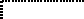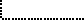Common Lisp the Language, 2nd EditionNext: Determining the Type Up: Type Specifiers Previous: Defining New Type

# 4.8. Type Conversion Function

The following function may be used to convert an object to an equivalent object of another type.

[Function]
coerce object result-type

The result-type must be a type specifier; the object is converted to an ``equivalent'' object of the specified type. If the coercion cannot be performed, then an error is signaled. In particular, (coerce x 'nil) always signals an error. If object is already of the specified type, as determined by typep, then it is simply returned. It is not generally possible to convert any object to be of any type whatsoever; only certain conversions are permitted:

• Any sequence type may be converted to any other sequence type, provided the new sequence can contain all actual elements of the old sequence (it is an error if it cannot). If the result-type is specified as simply array, for example, then (array t) is assumed. A specialized type such as string or (vector (complex short-float)) may be specified; of course, the result may be of either that type or some more general type, as determined by the implementation. Elements of the new sequence will be eql to corresponding elements of the old sequence. If the sequence is already of the specified type, it may be returned without copying it; in this, (coerce sequence type) differs from (concatenate type sequence), for the latter is required to copy the argument sequence. In particular, if one specifies sequence, then the argument may simply be returned if it already is a sequence.

```(coerce '(a b c) 'vector) => #(a b c)
```X3J13 voted in June 1989 (SEQUENCE-TYPE-LENGTH)   to specify that coerce should signal an error if the new sequence type specifies the number of elements and the old sequence has a different length.

X3J13 voted in March 1989 (CHARACTER-PROPOSAL)   to specify that if the result-type is string then it is understood to mean (vector character), and simple-string is understood to mean (simple-array character (*)).• Some strings, symbols, and integers may be converted to characters. If object is a string of length 1, then the sole element of the string is returned. If object is a symbol whose print name is of length 1, then the sole element of the print name is returned. If object is an integer n, then (int-char n) is returned. See character.

```(coerce "a" 'character) => #\a
```X3J13 voted in March 1989 (CHARACTER-PROPOSAL)   to eliminate int-char from Common Lisp. Presumably this eliminates the possibility of coercing an integer to a character, although the vote did not address this question directly.• Any non-complex number can be converted to a short-float, single-float, double-float, or long-float. If simply float is specified, and object is not already a float of some kind, then the object is converted to a single-float.

```(coerce 0 'short-float) => 0.0S0
(coerce 3.5L0 'float) => 3.5L0
(coerce 7/2 'float) => 3.5
```

• Any number can be converted to a complex number. If the number is not already complex, then a zero imaginary part is provided by coercing the integer zero to the type of the given real part. (If the given real part is rational, however, then the rule of canonical representation for complex rationals will result in the immediate re-conversion of the result from type complex back to type rational.)

```(coerce 4.5s0 'complex) => #C(4.5S0 0.0S0)
(coerce 7/2 'complex) => 7/2
(coerce #C(7/2 0) '(complex double-float))
=> #C(3.5D0 0.0D0)
```

• Any object may be coerced to type t.

```(coerce x 't) == (identity x) == x
```X3J13 voted in June 1988 (FUNCTION-TYPE)   to allow coercion of certain objects to the type function:

• A symbol or lambda-expression can be converted to a function. A symbol is coerced to type function as if by applying symbol-function to the symbol; an error is signaled if the predicate fboundp is not true of the symbol or if the symbol names a macro or special form. A list x whose car is the symbol lambda is coerced to a function as if by execution of (eval `#',x), that is, of (eval (list 'function x)).Coercions from floating-point numbers to rationals and from ratios to integers are purposely not provided because of rounding problems. The functions rational, rationalize, floor, ceiling, truncate, and round may be used for such purposes. Similarly, coercions from characters to integers are purposely not provided; char-code or char-int may be used explicitly to perform such conversions.Next: Determining the Type Up: Type Specifiers Previous: Defining New Type

AI.Repository@cs.cmu.edu